# Champagne Portraits of Complex Functions

Lots of tiny bubbles.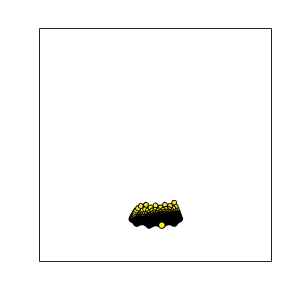### Contents

#### Domain

The basic domain is the quadruple unit square,

$$\max{(|x|,|y|)} \le 1, \ z = x+iy$$

#### Colors

I could use the HSV colormap.

   hsv_bubbles(@(z) z)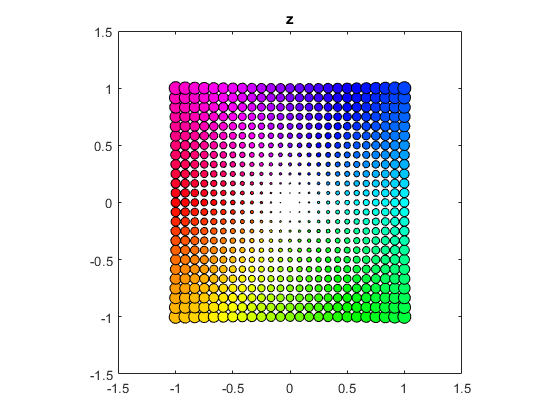But I prefer "periodic parula", the parula colormap concatenated with its reverse, [parula; flipud(parula)].

   bubbles(@(z) z)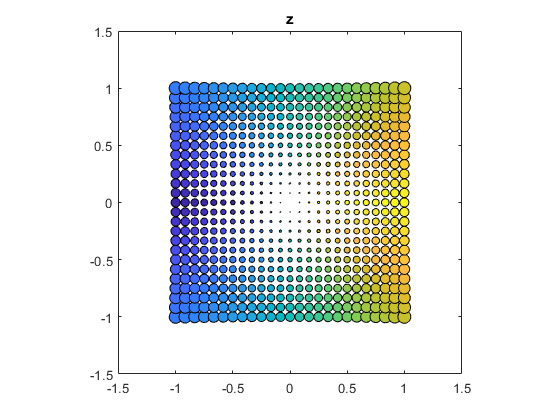#### z^2

   bubbles(@(z) z.^2)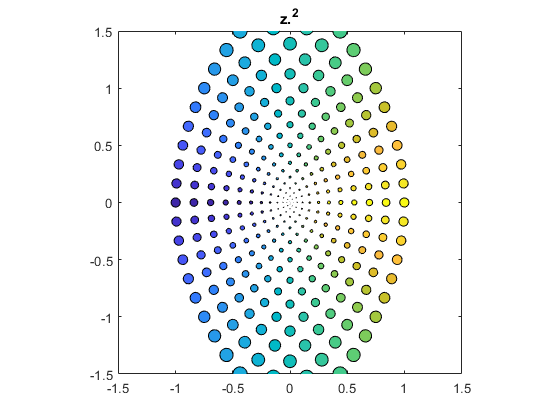#### z^3

   bubbles(@(z) z.^3)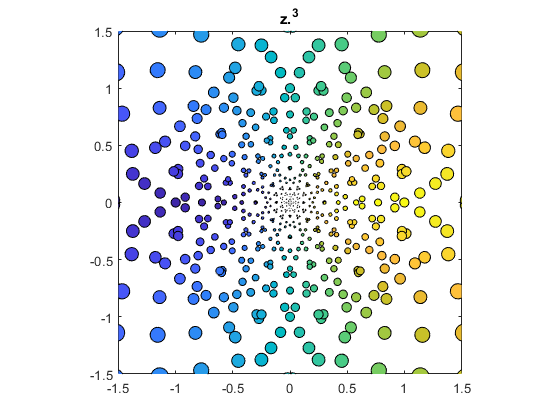#### z^9

   bubbles(@(z) z.^9)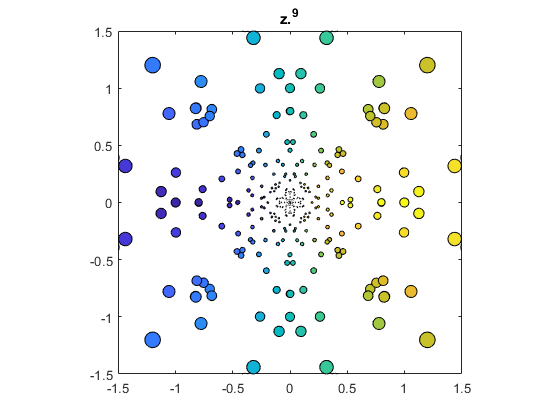#### 1/z

   bubbles(@(z) 1./z)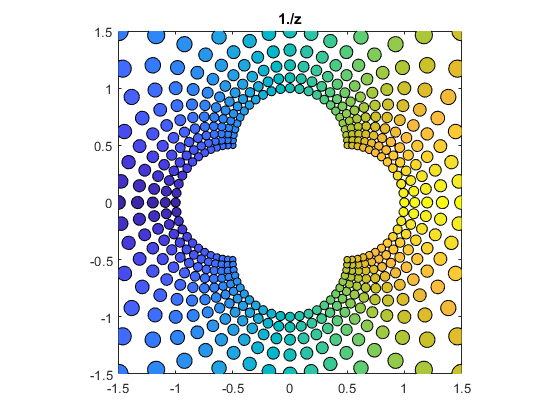#### sqrt(z)

A complex number has two square roots. One is in the right half plane.

   bubbles(@sqrt)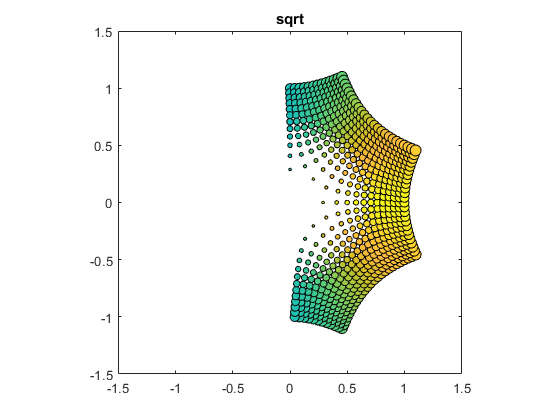#### -sqrt(z)

And the other is in the left half plane.

   bubbles(@(z) -sqrt(z))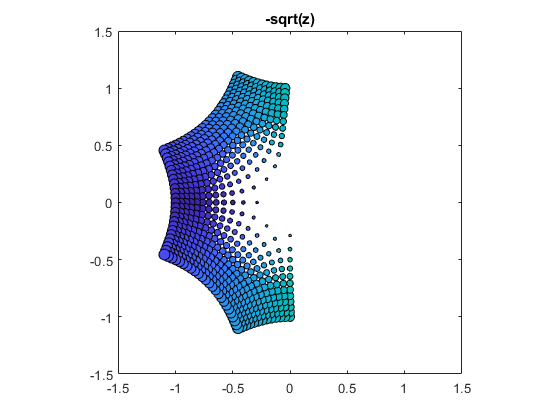#### sin(z)

   bubbles(@sin)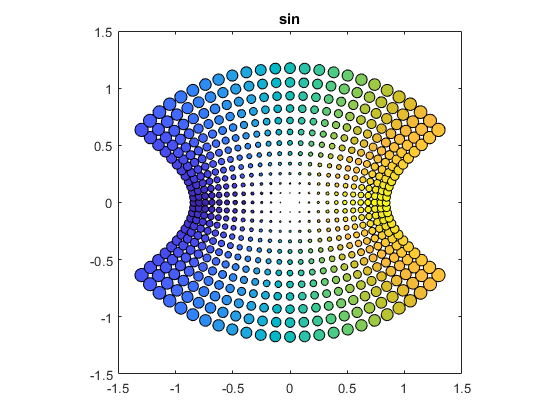#### cos(z)

   bubbles(@cos)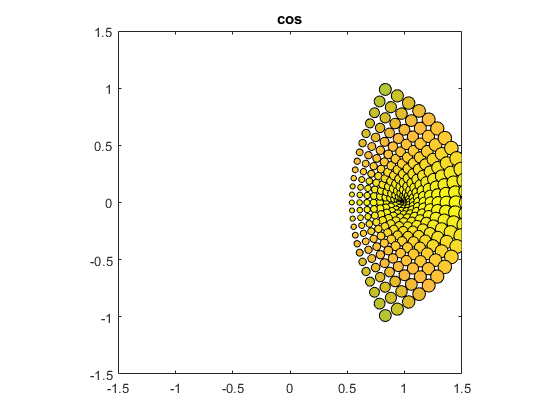#### tan(z)

   bubbles(@tan)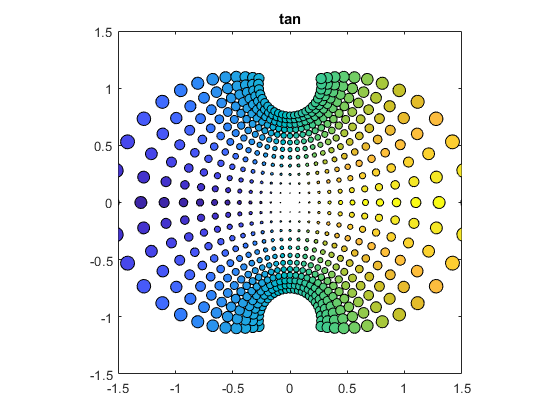#### cot(z)

   bubbles(@cot)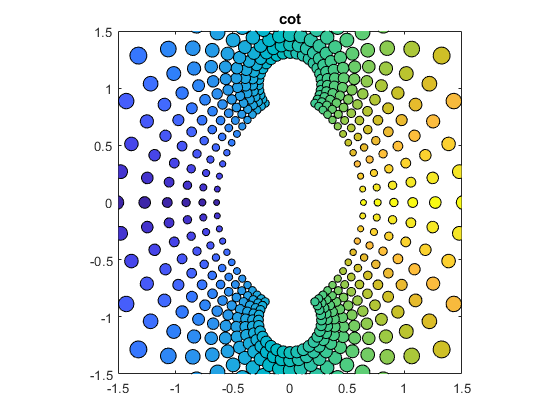#### exp(z)

These polar angles are between -1 and +1 radian.

   bubbles(@exp)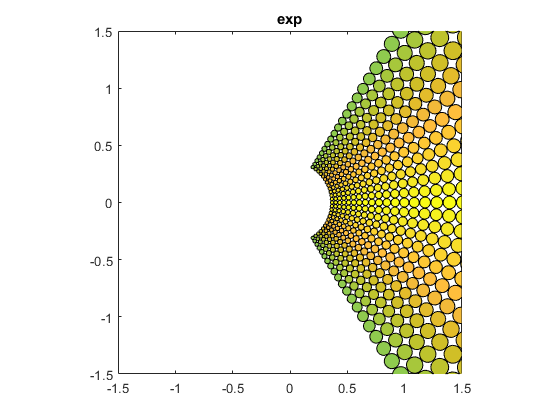#### exp(pi*z)/exp(pi)

These fill out the entire complex plane.

   bubbles(@(z) exp(pi*z)/exp(pi))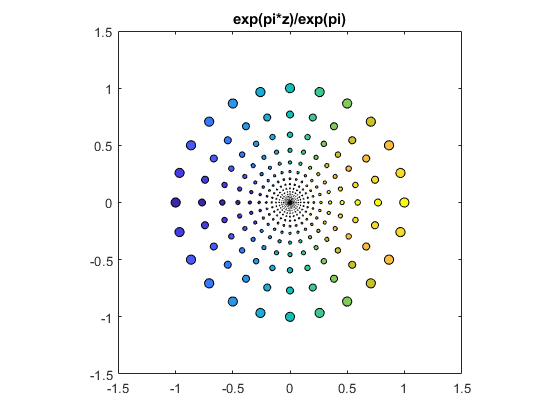#### log(z)

   bubbles(@log)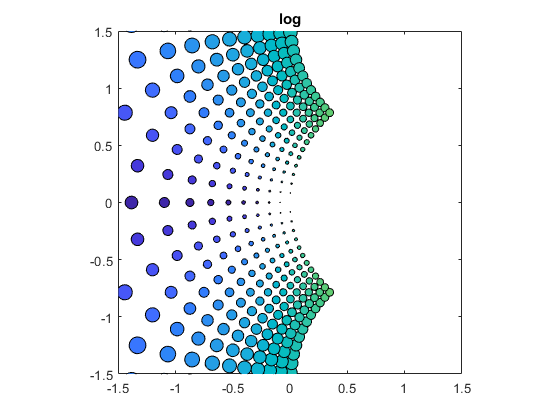#### z^3 - z

   bubbles(@(z) z.^3-z)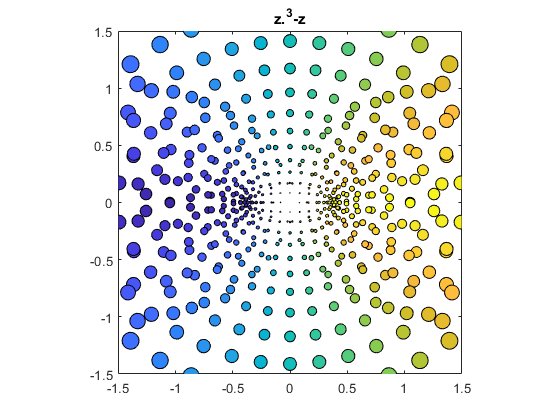#### .5/(z^5-z/5)

   bubbles(@(z) .5./(z.^5-z/5))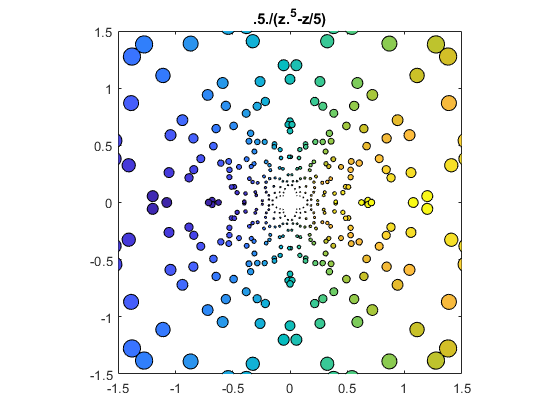#### exp(-1/(8 z^2)

   bubbles(@(z) exp(-1./(8*z.^2)))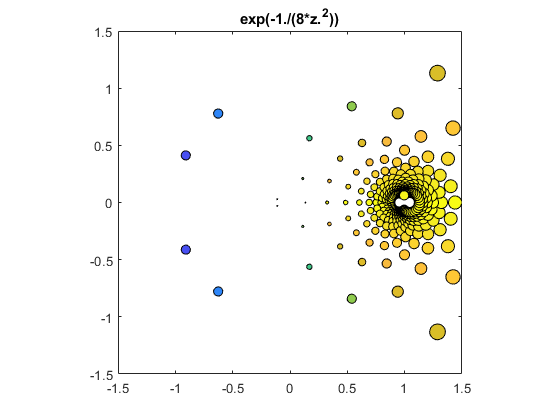#### Quiz

What is happening in the animation at the top of this post? If you think you know, or even if you just know part of the answer, submit a comment. I'll have some sort of prize for the first, or best, solution.

#### Code

Also available at https://blogs.mathworks.com/cleve/files/bubbles.m.

   type bubbles

function bubbles(F)
% bubbles. Color portrait of complex-valued function F(z),
% ex. bubbles(@sin)
%     bubbles(@(z) .5./(z.^5-z/5) )

if nargin < 1
F = @(z)z;
end

axis(1.5*[-1 1 -1 1])
axis square
box on
cla

m = 256;
colormap = [parula(m);flipud(parula(m))];

n = 25;
s = -1:2/(n-1):1;
[x,y] = meshgrid(s);
z = x + y*1i;
circle = exp((0:32)/16*pi*1i)/n;

w = F(z);
r = abs(w);
theta = angle(w)+pi;

scale = 20;
for k = 1:n
for j = 1:n
t = m*theta(k,j)/pi/scale;
idx = ceil(scale*t+realmin);
color = colormap(idx,:);
p = w(k,j) + r(k,j)*circle;
patch(real(p),imag(p),color)
end
end

titleF = char(F);
if (titleF(1) == '@')
titleF(1:4) = [];
end
title(titleF)
snapnow
end


Published with MATLAB® R2019b

|

### 댓글

댓글을 남기려면 링크 를 클릭하여 MathWorks 계정에 로그인하거나 계정을 새로 만드십시오.